Importance of Generalized Complex Sinusoids Next  |  Prev  |  Up  |  Top  |  Index  |  JOS Index  |  JOS Pubs  |  JOS Home  |  Search

### Importance of Generalized Complex Sinusoids

As a preview of things to come, note that one signal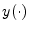4.15 is projected onto another signal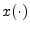using an inner product. The inner product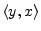computes the coefficient of projection4.16 of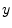onto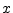. If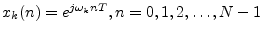(a sampled, unit-amplitude, zero-phase, complex sinusoid), then the inner product computes the Discrete Fourier Transform (DFT), provided the frequencies are chosen to be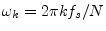. For the DFT, the inner product is specifically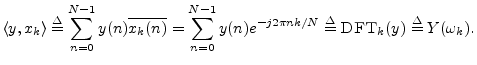Another case of importance is the Discrete Time Fourier Transform (DTFT), which is like the DFT except that the transform accepts an infinite number of samples instead of only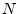. In this case, frequency is continuous, and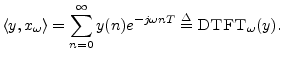The DTFT is what you get in the limit as the number of samples in the DFT approaches infinity. The lower limit of summation remains zero because we are assuming all signals are zero for negative time (such signals are said to be causal). This means we are working with unilateral Fourier transforms. There are also corresponding bilateral transforms for which the lower summation limit is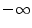. The DTFT is discussed further in §B.1.

If, more generally,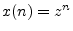(a sampled complex sinusoid with exponential growth or decay), then the inner product becomes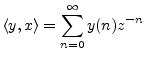and this is the definition of the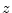transform. It is a generalization of the DTFT: The DTFT equals thetransform evaluated on the unit circle in theplane. In principle, thetransform can also be recovered from the DTFT by means of analytic continuation'' from the unit circle to the entireplane (subject to mathematical disclaimers which are unnecessary in practical applications since they are always finite).

Why have atransform when it seems to contain no more information than the DTFT? It is useful to generalize from the unit circle (where the DFT and DTFT live) to the entire complex plane (thetransform's domain) for a number of reasons. First, it allows transformation of growing functions of time such as growing exponentials; the only limitation on growth is that it cannot be faster than exponential. Secondly, thetransform has a deeper algebraic structure over the complex plane as a whole than it does only over the unit circle. For example, thetransform of any finite signal is simply a polynomial in. As such, it can be fully characterized (up to a constant scale factor) by its zeros in theplane. Similarly, thetransform of an exponential can be characterized to within a scale factor by a single point in theplane (the point which generates the exponential); since thetransform goes to infinity at that point, it is called a pole of the transform. More generally, thetransform of any generalized complex sinusoid is simply a pole located at the point which generates the sinusoid. Poles and zeros are used extensively in the analysis of recursive digital filters. On the most general level, every finite-order, linear, time-invariant, discrete-time system is fully specified (up to a scale factor) by its poles and zeros in theplane. This topic will be taken up in detail in Book II .

In the continuous-time case, we have the Fourier transform which projectsonto the continuous-time sinusoids defined by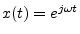, and the appropriate inner product is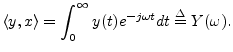Finally, the Laplace transform is the continuous-time counterpart of thetransform, and it projects signals onto exponentially growing or decaying complex sinusoids: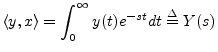The Fourier transform equals the Laplace transform evaluated along the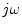axis'' in the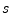plane, i.e., along the line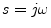, for which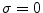. Also, the Laplace transform is obtainable from the Fourier transform via analytic continuation. The usefulness of the Laplace transform relative to the Fourier transform is exactly analogous to that of thetransform outlined above.

Next  |  Prev  |  Up  |  Top  |  Index  |  JOS Index  |  JOS Pubs  |  JOS Home  |  Search

[How to cite this work] [Order a printed hardcopy]